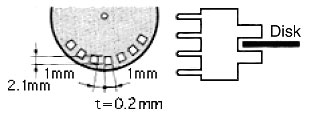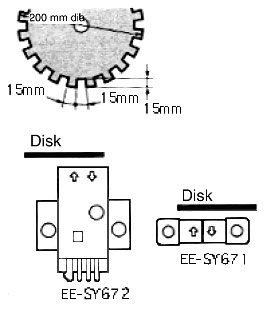# What is the response frequency?

The frequency at which an object satisfying specified conditions (size, transparency rate, reflection factor, sensing distance, and power supply voltage) can be repeatedly detected.

Example 1:

For EE-SX47[]/67[]-series (slot-type/non-modulated) Photomicrosensors, the response frequency is listed as 1 kHz (3 kHz average) in the response frequency column in the catalog's ratings and specifications. (See note 1.)

This means that OMRON ensures a response frequency of 1 kHz. This value takes product variation into account.

The average of the actual measured response frequencies is the value of 3 kHz listed in parentheses.

Use the average value as reference, but actually design the system using the ensured value of 1 kHz.

Note 1:
The response frequency above is measured for a disk rotated as in the following figure.Example 2:

For EE-SX671/672-series (reflective connector/non-modulated) Photomicrosensors, the response frequency is listed as 50 Hz (500 Hz average) in the response frequency column in the catalog's ratings and specifications. (See note 2.) The meanings of the ensured value and the average value are as explained above in example 1.

Note 2:
The response frequency above is measured for a disk rotated as in the following figure# Did this help answer your question?Thanks for the feedback! 🙏🏽